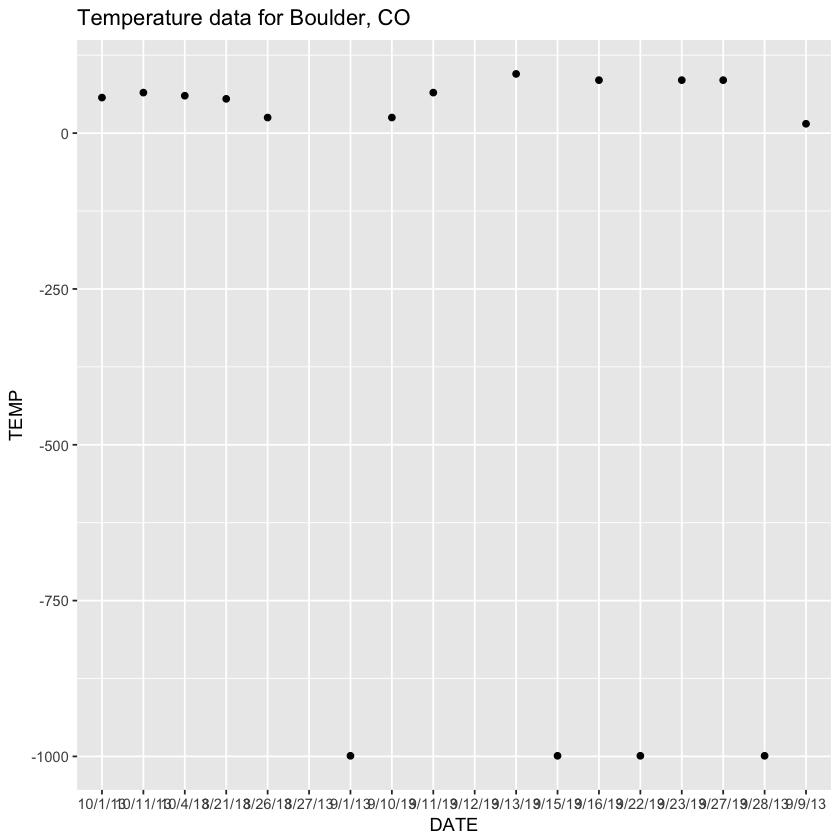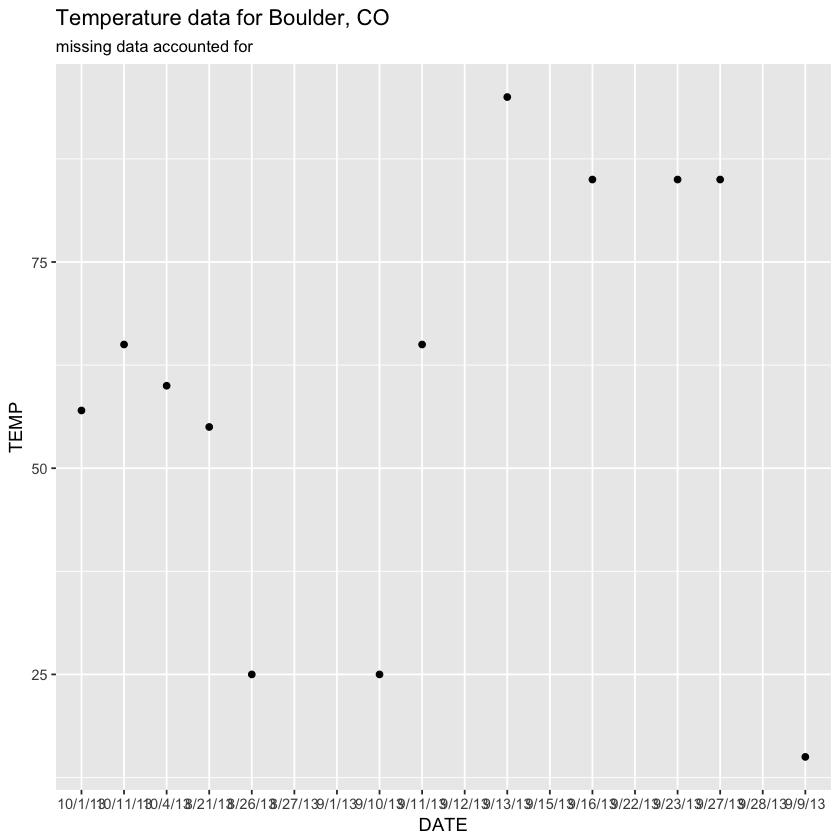# Lesson 5. How to Address Missing Values in R

This lesson covers how to work with no data values in R.

## Learning Objectives

At the end of this activity, you will be able to:

• Understand why it is important to make note of missing data values.
• Be able to define what a NA value is in R and how it is used in a vector.

## What You Need

You need R and RStudio to complete this tutorial. Also we recommend that you have an earth-analytics directory set up on your computer with a /data directory within it.

## Missing Data - No Data Values

Sometimes, your data are missing values. Imagine a spreadsheet in Microsoft Excel with cells that are blank. If the cells are blank, you don’t know for sure whether those data weren’t collected, or someone forgot to fill them in. To account for data that are missing (not by mistake) you can put a value in those cells that represents no data.

The R programming language uses the value NA to represent missing data values.


planets <- c("Mercury", "Venus", "Earth", "Mars", "Jupiter", "Saturn", "Uranus",
"Neptune", NA)


The default setting for most base functions that read data into R is to interpret NA as a missing value.

Let’s have a closer look at this using the boulder_precip data that you’ve used in the previous lessons. Please download the data again as there have been some changes made!

# download file
"data/boulder-precip.csv",
method = "libcurl")


Then you can open the data.

# import data but don't specify no data values - what happens?
boulder_precip <- read.csv(file = "data/boulder-precip.csv")

str(boulder_precip)
## 'data.frame':	18 obs. of  4 variables:
##  $ID : int 756 757 758 759 760 761 762 763 764 765 ... ##$ DATE  : chr  "8/21/13" "8/26/13" "8/27/13" "9/1/13" ...
##  $PRECIP: num 0.1 0.1 0.1 0 0.1 1 2.3 9.8 1.9 1.4 ... ##$ TEMP  : int  55 25 NA -999 15 25 65 NA 95 -999 ...


In the example below, note how a mean value is calculated differently depending upon on how NA values are treated when the data are imported.

# view mean values
mean(boulder_precip$PRECIP) ##  1.056 mean(boulder_precip$TEMP)
##  NA


Notice that you are able to calculate a mean value for PRECIP but TEMP returns a NA value. Why? Let’s plot your data to figure out what might be going on.

library(ggplot2)
# are there values in the TEMP column of your data?
boulder_precip$TEMP ##  55 25 NA -999 15 25 65 NA 95 -999 85 -999 85 85 ##  -999 57 60 65 # plot the data with ggplot ggplot(data = boulder_precip, aes(x = DATE, y = TEMP)) + geom_point() + labs(title = "Temperature data for Boulder, CO") ## Warning: Removed 2 rows containing missing values (geom_point).Looking at your data, it appears as if you have some extremely large negative values hovering around -1000. However why did your mean return NA? When performing mathematical operations on numbers in R, most functions will return the value NA if the data you are working with include missing or nodata values. Returning NA values allows you to see that you have missing data in your dataset. You can then decide how you want to handle the missing data. Youcan add the argument na.rm=TRUE to calculate the result while ignoring the missing values. heights <- c(2, 4, 4, NA, 6) mean(heights) ##  NA max(heights) ##  NA mean(heights, na.rm = TRUE) ##  4 max(heights, na.rm = TRUE) ##  6  Let’s try to add the na.rm argument to your code mean calculation on the temperature column above. # calculate mean usign the na.rm argument mean(boulder_precip$PRECIP)
##  1.056
mean(boulder_precip$TEMP, na.rm = TRUE) ##  -204.9  Data Tip: The functions, is.na(), na.omit(), and complete.cases() are all useful for figuring out if your data has assigned (NA) no-data values. See below for examples. So now you have successfully calculated the mean value of both precipitation and temperature in your spreadsheet. However does the mean temperature value (-204.9375 make sense looking at the data? It seems a bit low - you know that there aren’t temperature values of -200 here in Boulder, Colorado! Remembering the plot above you noticed that you had some values that were close to -1000. Looking at the summary below you see the exact minimum value is -999. # calculate mean usign the na.rm argument summary(boulder_precip$TEMP, na.rm = TRUE)
##    Min. 1st Qu.  Median    Mean 3rd Qu.    Max.    NA's
##    -999    -238      56    -205      70      95       2


## Finding & Assigning No Data Values

Sometimes, you’ll find a dataset that uses another value for missing data. In some disciplines, for example -999, is frequently used. If there are multiple types of missing values in your dataset, you can extend what R considers a missing value when it reads the file in using the “na.strings” argument.

Below use the na.strings argument on your data. Notice that you can tell R that there are several potential ways that your data documents nodata values.

You can provide R with a vector of missing date values as follows:

c("NA", " ", "-999")

Thus R will assign any calls with the values of nothing "", NA or -999 to NA. This should solve all of your missing data problems!

# import data but specify no data values - what happens?
boulder_precip_na <- read.csv(file = "data/boulder-precip.csv",
na.strings = c("NA", " ", "-999"))
boulder_precip_na$TEMP ##  55 25 NA NA 15 25 65 NA 95 NA 85 NA 85 85 NA 57 60 65  Does your new plot look better? # are there values in the TEMP column of your data? boulder_precip$TEMP
##     55   25   NA -999   15   25   65   NA   95 -999   85 -999   85   85
##  -999   57   60   65
# plot the data with ggplot
ggplot(data = boulder_precip_na, aes(x = DATE, y = TEMP)) +
geom_point() +
labs(title = "Temperature data for Boulder, CO",
subtitle = "missing data accounted for")
## Warning: Removed 6 rows containing missing values (geom_point).## Optional Challenge

• Question: Why, in the the example above did mean(boulder_precip$avg_temp) return a value of NA? • Question: Why, in the the example above did mean(boulder_precip$avg_temp, na.rm = TRUE) also return a value of NA?
# Extract those elements which are not missing values.
heights[!is.na(heights)]
##  2 4 4 6

# Returns the object with incomplete cases removed. The returned object is atomic.
na.omit(heights)
##  2 4 4 6
## attr(,"na.action")
##  4
## attr(,"class")
##  "omit"

# Extract those elements which are complete cases.
heights[complete.cases(heights)]
##  2 4 4 6


## Challenge Activity

• Question: Why does the following piece of code return a warning?
sample <- c(2, 4, 4, "NA", 6)
mean(sample, na.rm = TRUE)
## Warning in mean.default(sample, na.rm = TRUE): argument is not numeric or
## logical: returning NA
##  NA

• Question: Why does the warning message say the argument is not numeric?